The moments of a continuous probability distribution are often used to describe the shape of the probability density function (PDF). The first four moments (if they exist) are well known because they correspond to familiar descriptive statistics:

• The first raw moment is the mean of a distribution. For a random variable, this is the expected value. It can be positive, negative, or zero.
• The second central moment is the variance. The variance is never negative; in practice, the variance is strictly positive.
• The third standardized moment is the skewness. It can be positive, negative, or zero. A distribution that has zero skewness is symmetric.
• The fourth standardized moment is the kurtosis. Whereas the raw kurtosis, which is used in probability theory, is always positive, statisticians most often use the excess kurtosis. The excess kurtosis is 3 less than the raw kurtosis. Statisticians subtract 3 because the normal distribution has raw kurtosis of three, and researchers often want to compare whether the tail of a distribution is thicker than the normal distribution's tail (a positive excess kurtosis) or thinner than the normal distribution's tail (a negative excess kurtosis).

The purpose of this article is to point out a subtle point. There are three common definitions of moments: raw moments, central moments, and standardized moments. The adjectives matter. When you read a textbook, article, or software documentation, you need to know which definition the author is using.

### A cautionary tale

If you re-read the previous list, you will see that it is traditional to use the RAW moment for the mean, the CENTRAL moment for the variance, the STANDARDIZED moment for the skewness, and the STANDARDIZED moment (sometimes subtracting 3) for the kurtosis. However, researchers can report other quantities. Recently, I read a paper in which the author reported formulas for the third and fourth moments of a distribution. I assumed that the author was referring to the standardized moments, but when I simulated data from the distribution, my Monte Carlo estimates for the skewness and excess kurtosis did not agree with the author's formulas. After many hours of checking and re-checking the formulas and my simulation, I realized that the author's formulas were for the central moments (not standardized). After I converted the formulas to report standardized moments (and the excess kurtosis), I was able to reconcile the published results and my Monte Carlo estimates.

### Definitions of raw moments

For a continuous probability distribution for density function f(x), the nth raw moment (also called the moment about zero) is defined as
$\mu_n^\prime = \int_{-\infty}^{\infty} x^n f(x)\, dx$
The mean is defined as the first raw moment. Higher-order raw moments are used less often. The superscript on the symbol $\mu_n^\prime$ is one way to denote the raw moment, but not everyone uses that notation. For the first raw moment, both the superscript and the subscript are often dropped, and we use $\mu$ to denote the mean of the distribution.

For all moments, you should recognize that the moments might not exist. For example, the Student t distribution with ν degrees of freedom does not have finite moments of order ν or greater. Thus, you should mentally add the phrase "when they exist" to the definitions in this article.

### Definitions of central moments

The nth central moment for a continuous probability distribution with density f(x) is defined as
$\mu_n = \int_{-\infty}^{\infty} (x - \mu)^n f(x)\, dx$
where $\mu$ is the mean of the distribution. The most famous central moment is the second central moment, which is the variance. The second central moment, $\mu_2$ is usually denoted by $\sigma^2$ to emphasize that the variance is a positive quantity.

Notice that the central moments of even orders (2, 4, 6,...) are always positive since they are defined as the integral of positive quantities. It is easy to show that the first central moment is always 0.

The third and fourth central moments are used as part of the definition of the skewness and kurtosis, respectively. These moments are covered in the next section.

### Definitions of standardized moments

The nth standardized moment is defined by dividing the nth central moment by the nth power of the standard deviation:
${\tilde \mu}_n = \mu_n / \sigma^n$
For the standardized moments, we have the following results:

• The first standardized moment is always 0.
• The second standardized moment is always 1.
• The third standardized moment is the skewness of the distribution.
• The fourth standardized moment is the raw kurtosis of the distribution. Because the raw kurtosis of the normal distribution is 3, it is common to define the excess kurtosis as ${\tilde \mu}_n - 3$.

A distribution that has a negative excess kurtosis has thinner tails than the normal distribution. An example is the uniform distribution. A distribution that has a positive excess kurtosis has fatter tails than the normal distribution. An example is the t distribution. For more about kurtosis, see "Does this kurtosis make my tail look fat?"

### Moments for discrete distributions

Similar definitions exist for discrete distributions. Technically, the moments are defined by using the notion of the expected value of a random variable. Loosely speaking, you can replace the integrals by summations. For example, if X is a discrete random variable with a countable set of possible values {x1, x2, x3,...} that have probability {p1, p2, p3, ...} of occurring (respectively), then the raw nth moment for X is the sum
$E[X^n] = \sum_i x_i^n p_i$
and the nth central moment is
$E[(X-\mu)^n] = \sum_i (x_i-\mu)^n p_i$

### Summary

I almost titled this article, "Will the real moments please stand up!" The purpose of the article is to remind you that "the moment" of a probability distribution has several possible interpretations. You need to use the adjectives "raw," "central," or "standardized" to ensure that your audience knows which moment you are using. Conversely, when you are reading a paper that discusses moments, you need to determine which definition the author is using.

The issue is complicated because the common descriptive statistics refer to different definitions. The mean is defined as the first raw moment. The variance is the second central moment. The skewness and kurtosis are the third and fourth standardized moments, respectively. When using the kurtosis, be aware that most computer software reports the excess kurtosis, which is 3 less than the raw kurtosis.

The post Definitions of moments in probability and statistics appeared first on The DO Loop.

Monotonic transformations occur frequently in math and statistics. Analysts use monotonic transformations to transform variable values, with Tukey's ladder of transformations and the Box-Cox transformations being familiar examples. Monotonic distributions figure prominently in probability theory because the cumulative distribution is a monotonic increasing function. For a continuous distribution that is strictly monotonic, the quantile function is also monotonic.

In a recent project, I needed to determine whether a certain function is monotonic increasing. Because the function is only known at a finite sequence of values, the reduced problem is to determine whether a sequence is increasing. I have previously shown that you can use the DIF function in SAS to test for an increasing sequence. This article shows how to apply the method to the problem of deciding whether a function is increasing.

### What is a monotone sequence?

There are two types of monotonicity. In a weakly monotonic sequence, adjacent terms can be equal. That is, the difference between adjacent terms is allowed to be 0. In a strongly monotonic sequence (also called strictly monotonic), adjacent terms cannot be equal. For a strictly increasing sequence, the difference between adjacent terms is always positive. For a strictly decreasing sequence, the difference between adjacent terms is always negative. By itself, the term monotonic means the sequence is either increasing or decreasing.

Thus, there are four different tests for monotonicity. You can test whether a sequence is weakly increasing, strictly increasing, weakly decreasing, or strictly decreasing.

### Test for monotone increasing in SAS

The following SAS/IML module evaluates a vector and tests whether the elements are increasing. It uses the DIF function to produce a vector that contains the difference between adjacent elements of the input vector. That is, if x = {x1, x2, x3, ..., xn} is the input vector, then d = diff(x,1,1) is the vector {x2-x1, x3-x2, ..., xn-x[n-1]}. The second argument specifies the lag. The third argument is a flag that specifies whether the result is padded with missing values. (See the Appendix for more information.) By default, the function tests for a weakly increasing sequence.

proc iml; /* Test whether a sequence of elements is monotonic increasing. Valid options are strict=0 : (Default) Return 1 if a sequence is nondecreasing strict=1 : Return 1 if a sequence is strictly increasing */ start IsIncr(_x, strict=0); x = colvec(_x); if nrow(x)=1 then return(1); /* one element is always monotonic! */ d = dif(x,1,1); /* lag=1; delete initial missing value */ if strict then return( all(d > 0) ); return( all(d >= 0) ); finish;   /* test whether sequences are increasing */ x = {0,2,2,2,6,7,9}; y = {0,1,3,4,6,7,9}; z = {0,1,3,4,2,7,9}; b1 = IsIncr(x); /* test weakly increasing */ b2 = IsIncr(x, 1); /* test strictly increasing */ b3 = IsIncr(y, 1); /* test strictly increasing */ b4 = IsIncr(z); /* test weakly increasing */   print b1 b2 b3 b4;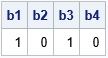The IsIncr function is called four times:

1. The first call returns the value 1 because the elements of x are weakly monotonic (nondecreasing).
2. The second call returns the value 0 because the elements of x are not strictly increasing.
3. The third call returns the value 1 because the elements of y are strictly increasing.
4. The fourth call returns the value 0 because the elements of z are not monotonic.

### Test for monotone decreasing in SAS

If a sequence {x1, x2, x3, ...} is monotone increasing, then the sequence obtained by multiplying each element by -1 is monotone decreasing. Therefore, it is trivial to write a function that tests whether a sequence is decreasing: simply test whether the negative of the sequence is increasing! This is accomplished by the following SAS/IML function:

/* Test whether a sequence of elements is monotonic decreasing. strict=0 : (Default) Return 1 if a sequence is nonincreasing strict=1 : Return 1 if a sequence is strictly decreasing */ start IsDecr(x, strict=0); return IsIncr(-x, strict); finish;   /* test whether sequence is increasing */ u = {9,8,7,7,6,2,0}; b5 = IsDecr(u); /* test weakly decreasing */ b6 = IsDecr(u, 1); /* test strictly decreasing */ print b5 b6;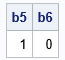The sequence is weakly decreasing but not strictly decreasing. The first call to the IsDecr function returns 1. The second call returns 0.

### An application: Test whether a transformation is monotonic

I used the IsIncr function to address a general question: Can you detect whether an unknown univariate function is monotonic increasing?

In a recent project, I was modeling the cumulative distribution function (CDF) of an unknown continuous distribution. By definition, a CDF must be increasing, but for some values of the parameters, the model is not an increasing function. I needed to identify these "infeasible" parameter values in a quick and reliable manner.

Mathematically, an increasing function, F, has the property that for every increasing sequence, {x_i}, the image of that sequence, {F(x_i)}, is also increasing. Numerically, you can generate an increasing sequence in the domain and ask whether the image of that sequence is also increasing.

Here's an example. Suppose you want to test whether the following functions are increasing:
F1(x) = (5 - 4*x) log( x/(1-x) )
F2(x) = (5 - 5.2*x) log( x/(1-x) )
The functions are defined on the domain (0, 1). The following program defines an increasing sequence on (0,1) and tests whether the image of the sequence is also increasing for F1 and for F2:

start Func1(x); return (5 - 4*x)#log( x/(1-x) ); finish; start Func2(x); return (5 - 5.2*x)#log( x/(1-x) ); finish;   dt = 0.005; x = do(dt, 1-dt, dt); /* increasing sequence on a fine grid */ y1 = Func1(x); /* image of sequence under F1 */ y2 = Func2(x); /* image of sequence under F2 */ b1 = IsIncr(y1); b2 = IsIncr(y2); print b1 b2;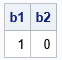The output indicates that the first function in increasing, but the second function is not. The following graph of the second function shows, indeed, that the function is not strictly increasing.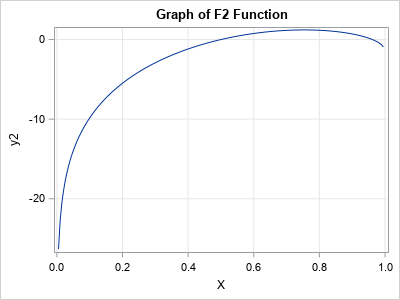The accuracy of this method depends on choosing a sequence on a fine grid of points in the domain. It assumes that the derivative of the function is bounded so that function cannot quickly increase and decrease on one of the small gaps between consecutive elements of the sequence.

### Summary

This article shows a way to test whether a function is monotonic on an interval. A common application is to test for an increasing function, but it is equally easy to test for a decreasing function. You can test whether the function is weakly increasing or strictly increasing.

These numerical tests evaluate a function at a finite set of points. Consequently, they can rule out the hypothesis of monotonicity, but they do not prove that the function is increasing everywhere. Nevertheless, these tests work well in practice if you choose the input sequence on a fine grid in the domain. If you have a mathematical bound on the derivative of the function, you can say more.

### Appendix: A useful option for the DIF function

By default, the DIF function in SAS/IML returns a vector that is the same size as the input vector. If k is the lag parameter, the first k elements are missing values. The differences between elements are contained in the elements k+1, k+2, and so forth. If you specify, the value 1 for the third option, the DIF function deletes the leading missing values. The result is a vector that has n – k elements, where n is the length of the input vector and k is the lag parameter.

For example, the following example passes in k=1 and deletes the leading missing value for the result:

t = {0,3,3,2,7}; /* input argument has 5 elements */ d = dif(t, 1, 1); /* LAG=1; delete leading missing value */ print d; /* result has 4 elements */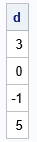The post A test for monotonic sequences and functions appeared first on The DO Loop.

It isn't easy to draw the graph of a function when you don't know what the graph looks like. To draw the graph by using a computer, you need to know the domain of the function for the graph: the minimum value (xMin) and the maximum value (xMax) for plotting the function. When you know a lot about the function, you can make well-informed choices for visualizing the graph. However, without knowledge, you might need several attempts before you discover the domain that best demonstrates the shape of the function. This trial-and-error approach is time-consuming and frustrating.

If the function is the density function for a probability distribution, there is a neat trick you can use to visualize the function. You can use quantiles of the distribution to determine the interval [xMin, xMax]. This article shows that you can set xMin to be an extreme-left quantile such as QUANTILE(0.01), and set xMin to be an extreme-right quantile such as QUANTILE(0.99). The values 0.01 and 0.99 are arbitrary, but in practice, these values provide good initial choices for many distributions. From looking at the resulting graph, you can often modify those numbers to improve the visualization.

### Choose a domain for the lognormal distribution

Here's an example. Suppose you want to graph the PDF or CDF of the standard lognormal distribution. What is the domain of the lognormal distribution? You could look it up on Wikipedia, but mathematically the 0.01 and 0.99 quantiles of the distribution provide an interval that contains 98% of the probability. Thus, is makes sense to initially visualize the function on this domain. The following SAS DATA step evaluates the PDF at 201 evenly spaced points on the interval [xMin, xMax]:

/* Choose xMin and xMax by using quantiles, then use equally spaced intervals in x */ data PDF; xMin = quantile("LogNormal", 0.01); /* p = 0.01 */ xMax = quantile("LogNormal", 0.99); /* p = 0.99 */ dx = (xMax - xMin) / 200; do x = xMin to xMax by dx; pdf = pdf("Lognormal", x); output; end; drop xMin xMax dx; run;   title "Visualize PDF by using Quantiles to Determine Domain"; proc sgplot data=PDF; series x=x y=PDF; run;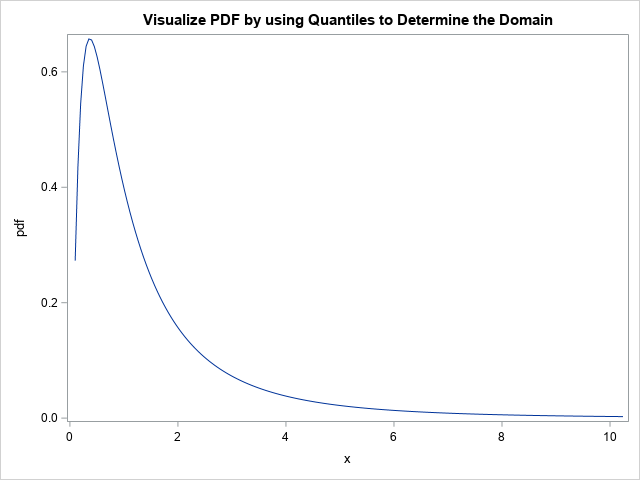This graph is pretty good, but not perfect. For the lognormal distribution, you might want xMin to move to the left so that the graph shows more of the shape of the left tail of the distribution. If you know that x=0 is the minimum value for the lognormal distribution, you could set xMin=0. However, you could also let the distribution itself decide the lower bound by decreasing the probability value used for the left quantile. You can change one number to re-visualize the graph. Just change the probability value from 0.01 to a smaller number such as 0.001 or 0.0001. For example, if you use 0.0001, the first statement in the DATA step looks like this:

xMin = quantile("LogNormal", 0.0001); /* p = 0.0001 */

With that small change, the graph now effectively shows the shape of the PDF for the lognormal distribution: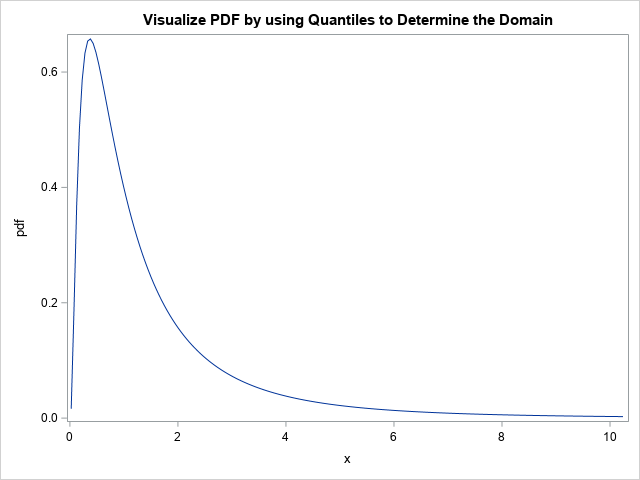### Visualize discrete densities

You can use the same trick to visualize densities or cumulative probabilities for a discrete probability distribution. For example, suppose you want to visualize the density for the Poisson(20) distribution. If you are familiar with the Poisson distribution, you might know that you need an interval that is symmetric about x=20 (the mean value), but how wide should the interval be? Instead of doing computations in your head, just use the quantile trick, as follows:

/* A discrete example: PDF = Poisson(20) */ data PMF; xMin = quantile("Poisson", 0.01, 20); /* p = 0.01 */ xMax = quantile("Poisson", 0.99, 20); /* p = 0.99 */ do x = xMin to xMax; /* x is integer-valued */ pmf = pdf("Poisson", x, 20); /* discrete probability mass function (PMF) */ output; end; drop xMin xMax; run;   title "Visualize Discrete PDF by using Quantiles to Determine the Domain"; proc sgplot data=PMF; vbar x / response=pmf; run;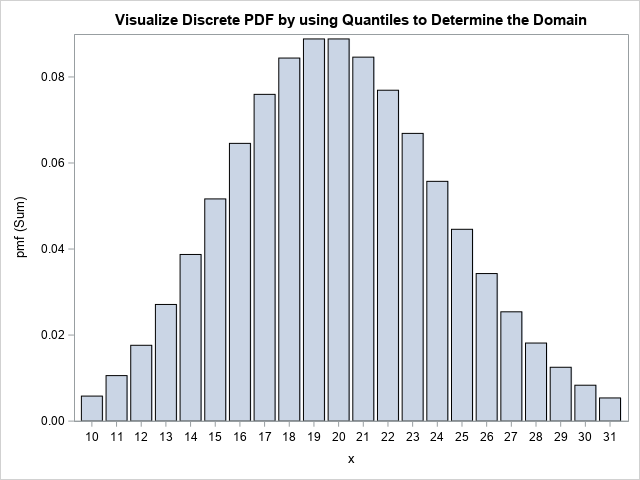Notice that the DATA step uses integer steps between xMin and xMax because the Poisson distribution is defined only for integer values of x. Notice also that I used a bar chart to visualize the density. Alternatively, you could use a needle chart. Regardless, the program automatically chose the interval [10, 31] as a representative domain. If you want to visualize more of the tails, you can use 0.001 and 0.999 as the input values to the QUANTILE call.

### Summary

This article shows a convenient trick for visualizing the PDF or CDF of a distribution function. You can use the quantile function to find quantiles in the left tail (xMin) and in the right tails (xMax) of the distribution. You can visualize the function by graphing it on the interval [xMin, xMax]. I suggest you use the 0.01 and 0.99 quantiles as an initial guess for the domain. You can modify those values if you want to visualize more (or less) of the tails of the distribution.

The post A tip for visualizing the density function of a distribution appeared first on The DO Loop.

A colleague was struggling to compute a right-tail probability for a distribution. Recall that the cumulative distribution function (CDF) is defined as a left-tail probability. For a continuous random variable, X, with density function f, the CDF at the value x is
F(x) = Pr(X ≤ x) = ∫ f(t) dt
where the integral is evaluated on the interval (-∞, x). That is, it is the area under the density function "to the left" of x. In contrast, the right-tail probability is the complement of this quantity. The right-tail probability is defined by
S(x) = Pr(X ≥ x) = 1 – F(x)
It is the area under the density function "to the right" of x.

The right-tail probability is also called the right-side or upper-tail probability. The function, S, that gives the right-tail probability is called the survival distribution function (SDF). Similarly, a quantile that is associated with a probability that is close to 0 or 1 might be called an extreme quantile. This article discusses why a naive computation of an extreme quantile can be inaccurate. It shows how to compute extreme probabilities and extreme quantiles by using special functions in SAS:

### Accurate computations of extreme probabilities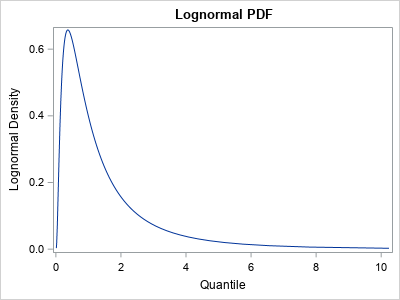My colleague contacted me to discuss some issues related to computing an extreme quantile for p ≈ 1. To demonstrate, I will use the standard lognormal distribution, whose PDF is shown to the right. (However, this discussion applies equally well to other distributions.) When p is small, the lognormal quantile is close to 0. When p is close to 1, the lognormal quantile is arbitrarily large because the lognormal distribution is unbounded.

The following SAS DATA step uses the QUANTILE function to compute extreme quantiles in the left and right tails of the lognormal distribution. The quantiles are associated with the probabilities p=1E-k and p=1 – 1E-k for k=8, 9, 10, ..., 15.

%let Distrib = Lognormal; data Have; do pow = -8 to -15 by -1; p = 10**pow; Lx = quantile("&Distrib", p); /* left-tail quantile: find x such that CDF(x) = p */ Rp = 1 - p; /* numerically, 1-p = 1 for p < MACEPS */ Rx = quantile("&Distrib", Rp); /* right-tail quantile: find x such that CDF(x) = 1-p */ output; end; format Rp 18.15; run;   proc print noobs; run;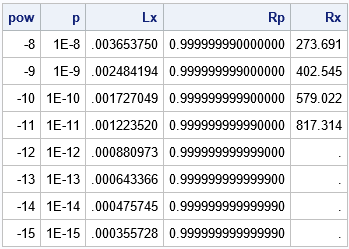The second column shows the probability, p, and the Lx column shows the corresponding left-tail quantile. For example, the first row shows that 1E-8 is the probability that a standard lognormal random variate has a value less than 0.00365. The Rp column shows the probability 1 – p. The Rx column shows the corresponding quantile. For example, the first row shows that 1 – 1E-8 is the probability that a standard lognormal random variate has a value greater than 273.691.

From experimentation, it appears that the QUANTILE function returns a missing value when the probability argument is greater than or equal to 1 – 1E-12. The SAS log displays a note that states
NOTE: Argument 2 to function QUANTILE('Normal',1) at line 5747 column 9 is invalid.
This means that the function will not compute extreme-right quantiles. Why? Well, I can think of two reasons:

• When p is very small, the quantity 1 – p does not have full precision. (This is called the "loss-of-precision problem" in numerical analysis.) In fact, if p is less than machine epsilon, the expression 1 – p EXACTLY EQUALS 1 in floating-point computations! Thus, we need a better way to specify probabilities that are close to 1.
• For unbounded distributions, the CDF function for an unbounded distribution is extremely flat for values in the right tail. Because the pth quantile is obtained by solving for the root of the function CDF(x) - p = 0, the computation to find an accurate extreme-right quantile is numerically imprecise for unbounded distributions.

### Compute extreme quantiles in SAS

The previous paragraph does not mean that you can't compute extreme-right quantiles. It means that you should use a function that is specifically designed to handle probabilities that are close to 1. The special function is the SQUANTILE function, which uses asymptotic expansions and other tricks to ensure that extreme-right quantiles are computed accurately. If x is the value returned by the SQUANTILE function, then 1 – CDF(x) = p. You can, of course, rewrite this expression to see that x satisfies the equation CDF(x) = 1 – p.

The syntax for the SQUANTILE function is almost the same as for the QUANTILE function. For any distribution, QUANTILE("Distrib", 1-p) = SQUANTILE("Distrib", p). That is, you add an 'S' to the function name and replace 1 – p with p. Notice that by specifying p instead of 1 – p, the right-tail probability can be specified more accurately.

The following SAS DATA step is a modification of the previous DATA step. It uses the SQUANTILE function to compute the extreme-right quantiles:

data Want; do pow = -8 to -15 by -1; p = 10**pow; Lx = quantile("&Distrib", p); /* find x such that CDF(x) = p */ Rp = 1 - p; Rx = squantile("&Distrib", p); /* find x such that 1-CDF(x)=p */ output; end; format Rp 18.15; drop pow; run;   proc print noobs; run;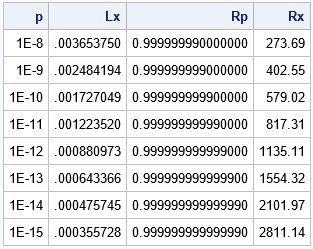The table shows that the SQUANTILE function can compute quantiles that are far in the right tail. The first row of the table shows that the probability is 1E-8 that a lognormal random variate exceeds 273.69. (Equivalently, 1 – 1E-8 is the probability that the variate is less than 273.69.) The last row of the table shows that 1E-15 is the probability that a lognormal random variate exceeds 2811.14.

### Compute extreme probabilities in SAS

When p is small, the "loss-of-precision problem" (representing 1 – p in finite precision) also affects the computation of the cumulative probability (CDF) of a distribution. That is, the expression CDF("Distrib", 1-p) might not be very accurate because 1 – p loses precision when p is small. To counter that problem, SAS provides the SDF function, which computes the survival distribution function (SDF). The SDF returns the right-tail quantile x for which 1 – CDF(x) = p. This is equivalent to finding the left-tail quantile that satisfies CDF(x) = 1 – p.

The following DATA step computes several one-sided probabilities. For each value of x, the program computes the probability that a random lognormal variate is greater than x:

%let Distrib = lognormal; data SDF; do x = 500 to 2500 by 500; /* extreme quantiles */ Rp = 1 - cdf("&Distrib", x); /* right-tail probability */ RpBetter = sdf("&Distrib", x); /* better computation of right-tail probability */ output; end; run;   proc print noobs; run;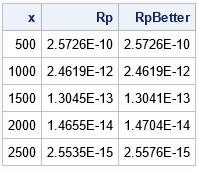The difference between the probabilities in the Rp and RpBetter columns are not as dramatic as for the quantiles in the previous section. That is because the quantity 1 – CDF(x) does not lose precision when x is large because CDF(x) ≈ 1. Nevertheless, you can see small differences between the columns, and the RpBetter column will tend to be more accurate when x is large.

### Summary

This article shows two tips for working with extreme quantiles and extreme probabilities. When the probability is close to 1, consider using the SQUANTILE function to compute quantiles. When the quantile is large, consider using the SDF function to compute probabilities.

The post Tips for computing right-tail probabilities and quantiles appeared first on The DO Loop.

It is important to be able to detect whether a numerical matrix is symmetric. Some operations in linear algebra require symmetric matrices. Sometimes, you can use special algorithms to factor a symmetric matrix. In both cases, you need to test a matrix for symmetry.

A symmetric matrix must be square. Mathematically, we say that an n x n matrix, A, is symmetric if A = AT. In terms of the elements of A, the matrix is symmetric if A[i,j] = A[j,i] for all 1 < i < j ≤ n.

### How to test a numerical matrix for symmetry

In numerical linear algebra, you should usually avoid testing floating-point numbers for equality. Instead, ask whether the difference | A[i,j] - A[j,i] | is smaller than some value, δ. But what small value should you choose for δ? Although a value such as δ = 1E-14 is suitable for many matrices, symmetry should not depend on the scaling of the matrix. In other words, the test for the matrices A and k*A should give the same results for any nonzero scalar, k.

One way to ensure that the scale of the matrix does not affect the result is to operate on a standardized matrix. You can use the elements of A to determine the scale factor. Let c = max(|A[i,j]|) for 1 ≤ i, j ≤ n. Define δ = c*sqrt(ε), where ε is machine precision. In SAS, you can use the CONSTANT('MACEPS') and CONSTANT('SQRTMACEPS') function calls to obtain the value of ε and sqrt(ε), respectively. SAS uses 2.22E-16 for machine precision, so sqrt(ε) ≈ 1.5e-8.

In summary, a good test for symmetry is to test whether all pairwise differences satisfy the equation | A[i,j] - A[j,i] | < c*sqrt(ε), where c = max(|A[i,j]|). This is equivalent to scaling A so that the largest element has unit magnitude and comparing the scaled differences with sqrt(ε).

In a matrix language such as SAS/IML, you can easily write a function that tests for symmetry, as follows:

proc iml;   start TestSym(A); if nrow(A) ^= ncol(A) then return(0); /* A is not square */ c = max(abs(A)); sqrteps = constant('SqrtMacEps'); return( all( abs(A-A) < c*sqrteps ) ); finish;

### Examples of testing a matric for symmetry

With that definition, let's see whether the function works on a few example matrices. The first example is a symmetric matrix. Let's see whether the test returns 1 (true) for the matrix and for various scalings of the matrix:

/* A is symmetric. TestSym(A) should return 1 (true). */ A = {1 2 3 -4 5, 2 3 2 3 3, 3 2 5 -1 0, -4 3 -1 4 -2, 5 3 0 -2 1 }; b1 = TestSym(A); b2 = TestSym(1E6 * A); b3 = TestSym(1E-8 * A); print b1 b2 b3;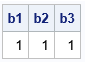Success. The TestSym function correctly detects that the matrix is symmetric. Various scalings of the matrix are also deemed symmetric.

What happens if we break the symmetry by making a tiny change to the (3,5) element?

/* break the symmetry by changing an off-diagonal element */ A[3,5] = 1e-6; d1 = TestSym(A); d2 = TestSym(1E6 * A); d3 = TestSym(1E-8 * A); print d1 d2 d3;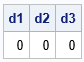For this matrix, the magnitude of the largest element is c=5. The perturbation to the matrix is 1e-6, which is less than c*sqrt(ε). Accordingly, the test detects that the perturbed matrix and its scalings are not symmetric. If you rerun the previous statements but use a smaller perturbation such as A[3,5] = 1e-9, the test will declare that the matrix is "numerically symmetric." Tiny differences between A[i,j] and A[j,i] are ignored, where "tiny" is relative to the magnitude of the largest element of A.

### Make a symmetric matrix

There are times when you might want to form a matrix that is symmetric from a matrix that is nearly symmetric. An easy way to do that is to use the matrix B = (A + AT)/2, as follows:

B = (A + A)/2; /* B is always symmetric */ isSym = TestSym(B); print isSym;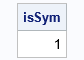For any square matrix, A, the matrix B = (A + AT)/2 is symmetric. I call the matrix B the symmetricization of A. An off-diagonal elements B[i,j] is the average of the corresponding elements A[i,j] and A[j,i].

### Summary

This article shows how to test a matrix for symmetry in numerical linear algebra. It uses the largest value of the matrix as a scale factor to standardize the computation. This technique is used in several SAS/IML functions that need to determine whether a matrix is symmetric.

### Test for skew symmetric matrix

Here is an exercise for the motivated reader. A square matrix, M, is skew-symmetric if it satisfies the equation M = -M. In other words, M[i,j] = -M[j,i] for all 1 < i, j ≤ n. Can you modify the TestSym function to test a matrix for a skew-symmetry to within some numerical precision? Hint: You only need to change one line!

The post Test for a symmetric matrix appeared first on The DO Loop.

It is important to be able to detect whether a numerical matrix is symmetric. Some operations in linear algebra require symmetric matrices. Sometimes, you can use special algorithms to factor a symmetric matrix. In both cases, you need to test a matrix for symmetry.

A symmetric matrix must be square. Mathematically, we say that an n x n matrix, A, is symmetric if A = AT. In terms of the elements of A, the matrix is symmetric if A[i,j] = A[j,i] for all 1 < i < j ≤ n.

### How to test a numerical matrix for symmetry

In numerical linear algebra, you should usually avoid testing floating-point numbers for equality. Instead, ask whether the difference | A[i,j] - A[j,i] | is smaller than some value, δ. But what small value should you choose for δ? Although a value such as δ = 1E-14 is suitable for many matrices, symmetry should not depend on the scaling of the matrix. In other words, the test for the matrices A and k*A should give the same results for any nonzero scalar, k.

One way to ensure that the scale of the matrix does not affect the result is to operate on a standardized matrix. You can use the elements of A to determine the scale factor. Let c = max(|A[i,j]|) for 1 ≤ i, j ≤ n. Define δ = c*sqrt(ε), where ε is machine precision. In SAS, you can use the CONSTANT('MACEPS') and CONSTANT('SQRTMACEPS') function calls to obtain the value of ε and sqrt(ε), respectively. SAS uses 2.22E-16 for machine precision, so sqrt(ε) ≈ 1.5e-8.

In summary, a good test for symmetry is to test whether all pairwise differences satisfy the equation | A[i,j] - A[j,i] | < c*sqrt(ε), where c = max(|A[i,j]|). This is equivalent to scaling A so that the largest element has unit magnitude and comparing the scaled differences with sqrt(ε).

In a matrix language such as SAS/IML, you can easily write a function that tests for symmetry, as follows:

proc iml;   start TestSym(A); if nrow(A) ^= ncol(A) then return(0); /* A is not square */ c = max(abs(A)); sqrteps = constant('SqrtMacEps'); return( all( abs(A-A) < c*sqrteps ) ); finish;

### Examples of testing a matric for symmetry

With that definition, let's see whether the function works on a few example matrices. The first example is a symmetric matrix. Let's see whether the test returns 1 (true) for the matrix and for various scalings of the matrix:

/* A is symmetric. TestSym(A) should return 1 (true). */ A = {1 2 3 -4 5, 2 3 2 3 3, 3 2 5 -1 0, -4 3 -1 4 -2, 5 3 0 -2 1 }; b1 = TestSym(A); b2 = TestSym(1E6 * A); b3 = TestSym(1E-8 * A); print b1 b2 b3;Success. The TestSym function correctly detects that the matrix is symmetric. Various scalings of the matrix are also deemed symmetric.

What happens if we break the symmetry by making a tiny change to the (3,5) element?

/* break the symmetry by changing an off-diagonal element */ A[3,5] = 1e-6; d1 = TestSym(A); d2 = TestSym(1E6 * A); d3 = TestSym(1E-8 * A); print d1 d2 d3;For this matrix, the magnitude of the largest element is c=5. The perturbation to the matrix is 1e-6, which is less than c*sqrt(ε). Accordingly, the test detects that the perturbed matrix and its scalings are not symmetric. If you rerun the previous statements but use a smaller perturbation such as A[3,5] = 1e-9, the test will declare that the matrix is "numerically symmetric." Tiny differences between A[i,j] and A[j,i] are ignored, where "tiny" is relative to the magnitude of the largest element of A.

### Make a symmetric matrix

There are times when you might want to form a matrix that is symmetric from a matrix that is nearly symmetric. An easy way to do that is to use the matrix B = (A + AT)/2, as follows:

B = (A + A)/2; /* B is always symmetric */ isSym = TestSym(B); print isSym;For any square matrix, A, the matrix B = (A + AT)/2 is symmetric. I call the matrix B the symmetricization of A. An off-diagonal elements B[i,j] is the average of the corresponding elements A[i,j] and A[j,i].

### Summary

This article shows how to test a matrix for symmetry in numerical linear algebra. It uses the largest value of the matrix as a scale factor to standardize the computation. This technique is used in several SAS/IML functions that need to determine whether a matrix is symmetric.

### Test for skew symmetric matrix

Here is an exercise for the motivated reader. A square matrix, M, is skew-symmetric if it satisfies the equation M = -M. In other words, M[i,j] = -M[j,i] for all 1 < i, j ≤ n. Can you modify the TestSym function to test a matrix for a skew-symmetry to within some numerical precision? Hint: You only need to change one line!

The post Test for a symmetric matrix appeared first on The DO Loop.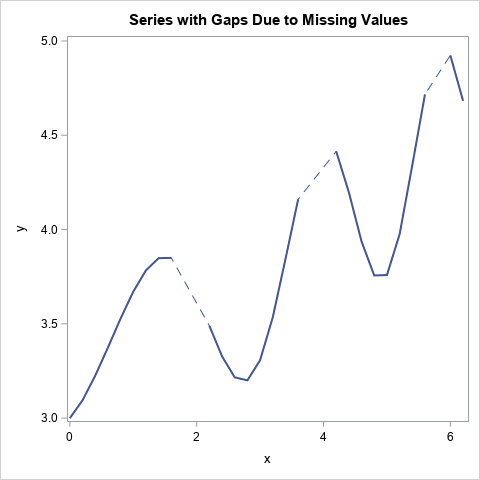A SAS programmer asked an interesting question: If data in a time series has missing values, can you plot a dashed line to indicate that the response is missing at some times?

A simple way to achieve this is by overlaying two lines. The first line (the "bottom" line in the overlay) is dashed. The second line (the "top" line in the overlay) is solid and uses the BREAK option to add gaps where the series has missing data. The result is shown to the left.

### Plotting gaps in the data

An important thing to remember about the SG graphics procedures in SAS is that points and lines are displayed in the same order as the statements that you specify. So, if you use two SERIES statements, the first line is plotted "on the bottom," and the second statement is plotted "on top."

A second important point is that you can use the BREAK option on the SERIES statement to force a break in the line for each missing value for the Y variable. The BREAK statement causes the line to appear as multiple line segments that do not "connect through" missing data. If you do not use the BREAK statement, the SERIES statement will connect each valid data point to the next valid data point.

You can therefore plot two lines. The bottom line is dashed and connects through missing values. The top line is solid and breaks at missing values. This is shown in the following call to PROC SGPLOT:

/* create data that has some missing Y values */ data Have; do x = 0 to 6.2 by 0.2; cnt + 1; y = 3 + x/4 + 0.5*sin(x**1.5); if cnt=10 | cnt=11 | cnt=20 | cnt=21 | cnt=30 | cnt=40 | cnt=41 then y = .; output; end; run;   title "Series with Gaps Due to Missing Values"; proc sgplot data=Have noautolegend; series x=x y=y / lineattrs=GraphData1(pattern=dash); series x=x y=y / BREAK lineattrs=GraphData1(pattern=solid thickness=2); run;

There might be times when you want to enhance the series plot by showing more information about the location of the nonmissing data. An easy way to do that is to use the MARKERS option to add markers to the graph. The markers are displayed only at locations for which both X and Y are nonmissing. A second way to visualize the locations of the nonmissing values is to add a fringe plot along the bottom of the line plot, as follows:

/* append the "fringe" data: the X value of points that have nonmissing Y value */ data Want; set Have Have(rename=(x=t) where=(y ^= .)); keep x y t; run;   title "Series and Fringe Plot"; proc sgplot data=Want noautolegend; series x=x y=y / lineattrs=GraphData1(pattern=dash); series x=x y=y / markers BREAK lineattrs=GraphData1(pattern=solid thickness=2); fringe t; run;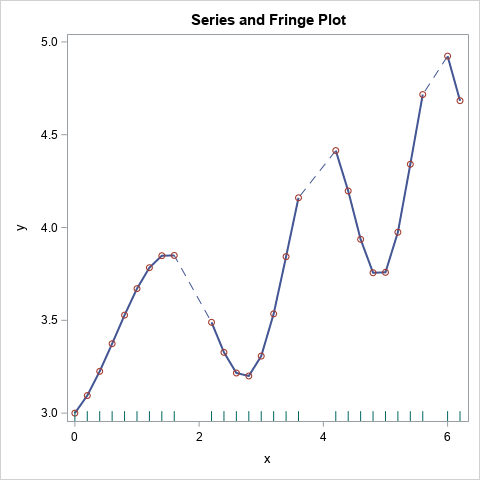This graph makes it easier to see the nonmissing values and the locations of the gaps in the data.

### Summary

This article shows a cool trick for using a dashed line to indicate that a time series has missing values. The trick is to overlay a dashed line and a solid line. By using the BREAK option on the solid line, the underlying dashed line shows through and visually indicates that missing values are present in the data.

The post A trick to plot a time series that has missing values appeared first on The DO Loop.

Last year, I wrote almost 100 posts for The DO Loop blog. My most popular articles were about data visualization, statistics and data analysis, and simulation and bootstrapping. If you missed any of these gems when they were first published, here are some of the most popular articles from 2021:

### SAS programming and data visualization

• Display the first or last observations in data: Whether your data are in a SAS data set or a SAS/IML matrix, this article describes how to display to print the top rows (and bottom rows!) of a rectangular data set.
• Customize titles in a visualization of BY groups: Have you ever use the BY statement to graph data across groups, such as Males/Females or Control/Experimental groups? If so, you might want to learn how to use the #BYVAR and #BYVAL keywords to customize the titles that appear on each graph.
• Reasons to prefer a horizontal bar chart: Bar charts are used to visualize the counts of categorical data. Vertical charts are used more often, but there are advantages to using a horizontal bar chart, especially if you are displaying many categories or categories that have long labels. This article shows how to create a horizontal bar chart in SAS and gives examples for which a horizontal chart is preferable.
• Why you should visualize distributions: It is common to report the means (of difference between means) for different groups in a study. However, means and standard deviations only tell part of the story. This article shows four examples where the mean difference between group scores is five points. However, when you plot the data for each example, you discover additional information about how the groups differ.

### Simulation and Bootstrapping

Since I am the guy who wrote the book on statistical simulation in SAS, it is not surprising that my simulation articles are popular. Simulation helps analysts understand expected values, sampling variation, and standard errors for statistics.

Did you resolve to learn something new in the New Year? Reading these articles requires some effort, but they provide tips and techniques that make the effort worthwhile. So, read (or re-read!) these popular articles from 2021. To ensure you don't miss a single article in 2022, consider subscribing to The DO Loop.

The post Top 10 posts from <em>The DO Loop</em> in 2021 appeared first on The DO Loop.

SAS/IML programmers often create and call user-defined modules. Recall that a module is a user-defined subroutine or function. A function returns a value; a subroutine can change one or more of its input arguments. I have written a complete guide to understanding SAS/IML modules, which contains may tips for working with SAS/IML modules. Among the tips are two that can trip up programmers who are new to the SAS/IML language:

In the years since I wrote those articles, SAS/IML introduced lists, which are a popular way to pass many arguments to a user-defined module. Lists and matrices behave similarly when passed to a module:

• If you modify a list in a module, the list is also changed in the calling environment.
• If you specify an expression such as [A, B], SAS/IML creates a temporary list.

This article shows a subroutine that takes a list and modifies the items in the list. It also looks at what happens if you pass in a temporary list.

### Review: Passing a matrix to a module

Before discussing lists, let's review the rules for passing a matrix to a user-defined module. The following user-defined subroutine doubles the elements for its matrix argument:

proc iml; /* Double the values in X. The matrix X is changed in the calling environment. */ start Double(X); X = 2*X; finish;   r1 = 1:3; r2 = 4:6; A = r1 // r2; /* each row of A is a copy. r1 and r2 do not share memory with A. */ run Double(A); print A;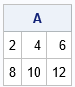As shown by the output, the elements of A are changed after passing A to the Double module. Notice also that although A was constructed from vectors r1 and r2, those vectors are not changed. They were used to initialize the values of A, but they do not share any memory with A.

Now suppose you want to double ONLY the first row of A. The following attempt does NOT double the first row:

A = r1 // r2; /* each row of A is a copy */ run Double( A[1,] ); /* NOTE: create temporary vector, which is changed but vanishes */ print A;

The result is not shown because the values of A are unchanged. The matrix is not changed because it was not sent into the module. Instead, a temporary matrix (A[1,]) was passed to the Double module. The values of the temporary matrix were changed, but that temporary matrix vanishes, so there is no way to access those modified values. (See the previous article for the correct way to double the first row.)

### Passing lists: The same rules apply

These same rules apply to lists, as I demonstrate in the next example. The following user-defined subroutine takes a list as an argument. It doubles every (numerical) item in the list. This modification affects the list in the calling environment.

/* Double the values of every numerical matrix in a list, Lst. Lst will be changed in the calling environment. */ start DoubleListItems(Lst); if type(Lst)^='L' then stop "ERROR: Argument is not a list"; nItems = ListLen(Lst); do i = 1 to nItems; if type(Lst$i)='N' then Lst$i = 2*Lst$i; /* the list is changed in the calling environment */ end; finish; A = {1 2 3, 4 5 6}; B = -1:1; L = [A, B]; /* each item in L is a copy */ run DoubleListItems(L);/* the copies are changed */ print (L$1)[label="L$1"], (L$2)[label="L\$2"];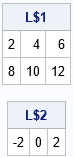As expected, the items in the list were modified by the DoubleListItems subroutine. The list is modified because we created a "permanent" variable (L) and passed it to the module. If you simply pass in the expression [A, B], then SAS/IML creates a temporary list. The module modifies the items in the temporary list, but you cannot access the modified values because the temporary list vanishes:

/* create temporary list. Items in the list are changed but the list vanishes */ run DoubleListItems( [A, B] ); /* no way to access the modified values! */

### Summary

This brief note is a reminder that SAS/IML creates temporary variables for expressions like X[1,] or [A, B]. In most cases, programmers do not need to think about this fact. However, it becomes important if you write a user-defined module that modifies one of its arguments. If you pass a temporary variable, the modifications are made to the temporary variable, which promptly vanishes after the call. To prevent unexpected surprises, always pass in a "permanent" variable to a module that modifies its arguments.

The post On passing a list to a SAS/IML module appeared first on The DO Loop.Have you ever heard of the DOLIST syntax? You might know the syntax even if you are not familiar with the name. The DOLIST syntax is a way to specify a list of numerical values to an option in a SAS procedure. Applications include:

• Specify the end points for bins of a histogram
• Specify percentiles to be output to a data set
• Specify tick marks for a custom axis on a graph
• Specify the location of reference lines on a graph
• Specify a list of parameters for an algorithm. Examples include smoothing parameters (the SMOOTH= option in PROC LOESS), sample sizes (the NTOTAL= option in PROC POWER), and initial guess for parameters in an optimization (the PARMS statement in PROC NLMIXED and PROC NLIN)

This article demonstrates how to use the DOLIST syntax to specify a list of values in SAS procedures. It shows how to use a single statement to specify individual values and also a sequence of values.

The DOLIST syntax enables you to write a single statement that specifies individual values and one or more sequences of values. The DOLIST syntax should be in the toolbox of every intermediate-level SAS programmer!

### The DOLIST syntax in the SAS DATA step

According to the documentation of PROC POWER, the syntax described in this article is sometimes called the DOLIST syntax because it is based on the syntax for the iterative DO loop in the DATA step.

The most common syntax for a DO loop is DO x = start TO stop BY increment. For example, DO x = 10 TO 90 BY 20; iterates over the sequence of values 10, 30, 50, 70, and 90. If the increment is 1, you can omit the BY increment portion of the statement. However, you can also specify values as a common-separated list, such as DO x = 10, 30, 50, 70, 90;, which generates the same values. What you might not know is that you can combine these two methods. For example, in the following DATA step, the values are specified by using two comma-separated lists and three sequences. For clarity, I have placed each list on a separate line, but that is not necessary:

/* the DOLIST syntax for a DO loop in the DATA step */ data A; do pctl = 5, /* individual value(s) */ 10 to 50 by 20, /* a sequence of values */ 54.3, 69.1, /* individual value(s) */ 80 to 90 by 5, /* another sequence */ 60 to 40 by -20; /* yet another sequence */ output; end; run;   proc print; run;

The output (not shown) is a list of values: 5, 10, 30, 50, 54.3, 69.1, 80, 85, 90, 60, 40. Notice that the values do not need to be in sorted order, although they often are.

The expressions to the right of the equal sign are what I mean by the "DOLIST syntax." You can use the same syntax to specify a list of options in many SAS procedures. When the SAS documentation says that an option takes a "list of values," you can often use a comma-separated list, a space-separated list, and the syntax start TO stop BY increment. (Or a combination of these expressions!) The following sections provide a few examples, but there are literally hundreds of options in SAS that support the DOLIST syntax!

Some procedures (for example, PROC SGPLOT) require the DOLIST values to be in parentheses. Consequently, I have adopted the convention of always using parentheses around DOLIST values, even if the parentheses are not strictly required. As far as I know, it is never wrong to put the DOLIST inside parentheses, and it keeps me from having to remember whether parentheses are required. The examples in this article all use parentheses to enclose DOLIST values.

### Histogram bins and percentiles

You can use the DOLIST syntax to specify the endpoints of bins in a histogram. For example, in PROC UNIVARIATE, the ENDPOINTS= option in the HISTOGRAM statement supports a DOLIST. Because histograms use evenly spaced bins, usually you will specify only one sequence, as follows:

proc univariate data=sashelp.cars; var weight; histogram weight / endpoints=(1800 to 7200 by 600); /* DOLIST sequence expression */ run;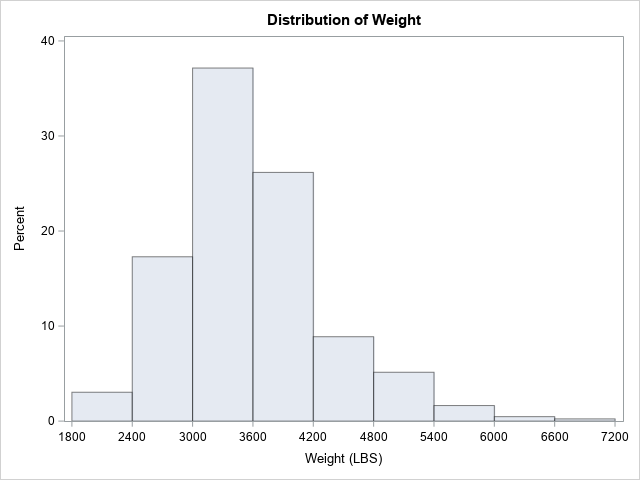You can also use the DOLIST syntax to specify percentiles. For example, the PCTLPTS= option on the OUTPUT statement enables you to specify which percentiles of the data should be written to a data set:

proc univariate data=sashelp.cars; var MPG_City; output out=UniOut pctlpre=P_ pctlpts=(50 75, 95 to 100 by 2.5); /* DOLIST */ run;

Notice that this example specifies both individual percentiles (50 and 75) and a sequence of percentiles (95, 97.5, 100).

### Tick marks and reference lines

The SGPLOT procedure enables you to specify the locations of tick marks on the axis of a graph. Most of the time you will specify an evenly spaced set of values, but (just for fun) the following example shows how you can use the DOLIST syntax to combine evenly spaced values and a few custom values:

title "Specify Ticks on the Y Axis"; proc sgplot data=sashelp.cars; scatter x=Weight y=Mpg_City; yaxis grid values=(10 to 40 by 5, 50 60); /* DOLIST; commas optional */ run;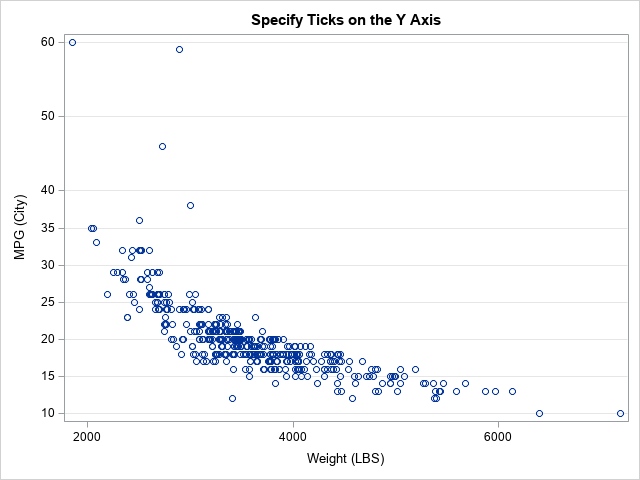As shown in the previous example, the GRID option on the XAXIS and YAXIS statements enables you to display reference lines at each tick location. However, sometimes you want to display reference lines independently from the tick marks. In that case, you can use the REFLINE statement, as follows:

title "Many Reference Lines"; proc sgplot data=sashelp.cars; scatter x=Weight y=MPG_City; refline (1800 to 6000 by 600, 7000) / axis=x; /* many reference lines */ run;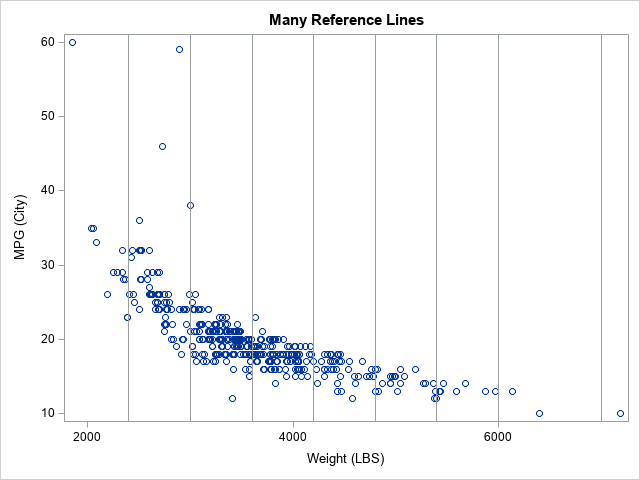### Statistical procedures

Many statistical procedures have options that support lists. In most cases, you can use the DOLIST syntax to provide values for the list.

I have already written about how to use the DOLIST syntax to specify initial guesses for the PARM statement in PROC NLMIXED and PROC NLIN. The documentation for the POWER procedure discusses how to specify lists of values and uses the term "DOLIST" in its discussion.

Some statistical procedures enable you to specify multiple parameter values, and the analysis is repeated for each parameter in the list. One example is the SMOOTH= option in the MODEL statement of the LOESS procedure. The SMOOTH= option specifies values of the loess smoothing parameter. The following call to PROC LOESS fits four loess smoothers to the data. The call to PROC SGPLOT overlays the smoothers on a scatter plot of the data:

proc loess data=sashelp.cars plots=none; model MPG_City = Weight / smooth=(0.1 to 0.5 by 0.2, 0.75); /* value-list */ output out=LoessOut P=Pred; run;   proc sort data=LoessOut; by SmoothingParameter Weight; run; proc sgplot data=LoessOut; scatter x=Weight y=MPG_City / transparency=0.9; series x=Weight y=Pred / group=SmoothingParameter curvelabel curvelabelpos=min; run;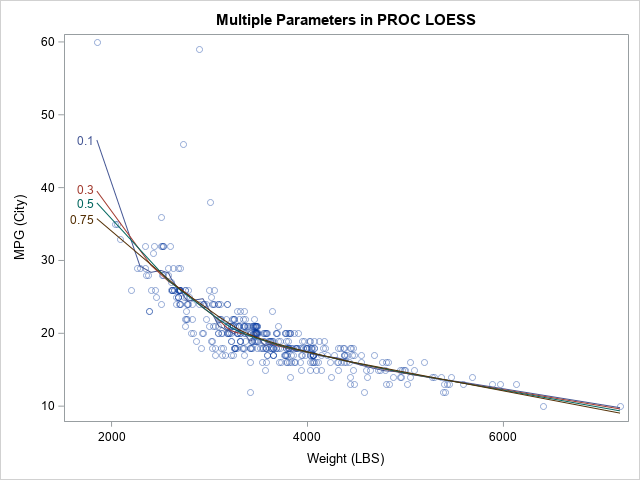### Summary

In summary, this article describes the DOLIST syntax in SAS, which enables you to simultaneously specify individual values and evenly spaced sequences of values. A sequence is specified by using the start TO step BY increment syntax. The DOLIST syntax is valid in many SAS procedures and in the DATA step. In some procedures (such as PROC SGPLOT), the syntax needs to be inside parentheses. For readability, you can use commas to separate individual values and sequences.

Many SAS procedures accept the special syntax even if it is not explicitly mentioned in the documentation. In the documentation for an option, look for terms such as value-list or numlist or value-1 <...value-n>, which indicate that the option supports the DOLIST syntax.

The post The DOLIST syntax: Specify a list of numerical values in SAS appeared first on The DO Loop.